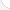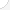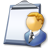Aerospace engineers Description: Perform a variety of engineering work in designing, constructing, and testing aircraft, missiles, and spacecraft. May conduct basic and applied research to evaluate adaptability of materials and equipment to aircraft design and manufacture. May recommend improvements in testing equipment and techniques.Source: Bureau of Labor Statistics, U.S. Department of Labor, Occupational Outlook Handbook, 2008-09 Edition at http://www.bls.gov/OCO/Complete Job Profile: http://www.bls.gov/oco/ocos027.htmSalary: \$50,001 or more per yearComments:Any career in any scientific or engineering fields will require both basic and advanced math. Without math to determine principles, calculate dimensions and limits, explore variations, prove concepts, etc. there would be no cell phones, TVs, stereos, video games, microwave ovens, computers, or virtually anything electronic. There would be no bridges, tunnels, roads, skyscrapers, automobiles, ships, planes, rockets or most things mechanical. There would be no metals beyond the common, like iron and copper, no plastics, no synthetics. In short, we'd most likely still be cave-dwellers without the use of math throughout the centuries and into the future. - richfro@juno.com (Rich Fro)

There are 66 math topics Aerospace engineers need to know.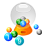Basic Math / Algebra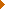FractionsDecimalsRatio and ProportionPercentCustomary MeasurementMetric MeasurementMeasurement ConversionBasic ProbabilityBasic StatisticsStatistical GraphingPowers and RootsOther Number BasesNegative NumbersScientific NotationBasic Problem Solving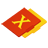First-Year AlgebraUsing FormulasLinear EquationsLinear InequalitiesOperations with PolynomialsRational ExpressionsCoordinate Graphing 2DLinear SystemsRadicalsQuadratic EquationsAlgebraic Representation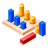GeometryBasic TerminologyAngle MeasurementParallel LinesQuadrilateralsSimilarityGeometric MeanPythagorean TheoremRight Triangle TrigonometryCirclesConstructionsAreaVolumeTransformationsMake/Use 3D Drawings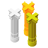Second-Year Algebra / TrigonometryFunctionsVariationImaginary NumbersPolynomial EquationsLogarithmsMatricesCoordinate Graphing 3DAdvanced ProbabilityAdvanced StatisticsConic SectionsNon-Linear SystemsTrigonometric/Circular FunctionsGraphs of Trigonometric FunctionsTrigonometric IdentitiesTrigonometric Equations/InversesOblique TrianglesPolar Coordinates/GraphsVectors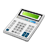Other TopicsCalculus and Higher MathBasic Calculator UseScientific Calculator UseComputer UseGroup Problem SolvingMental MathInductive/Deductive ReasoningMath CommunicationsMathematical Modeling